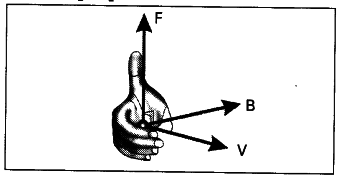# Explain magnetic force on moving charge and current carrying wire?

a) TV Screen Activity :

1. Take a bar magnet and bring it near the TV screen.
2. Then the picture on the screen is distorted.
3. Here the distortion is due to the motion of the electrons reacting the screen are affected by the magnetic field.
4. Now move the bar magnet away from the screen.
5. Then the picture on the screen stabilizes.
6. This must be due to the fact that the magnetic field exerts a force on moving charges. This force is called magnetic force.
7. The magnitude of the force is F = Bqv where B is magnetic induction, ‘q’ is the charge and v is the velocity of the charged particle.
b) Procedure :
8. Take a bar magnet near to the TV screen. We observe that electrons are affected by the field produced by the bar magnet. Then the picture is disturbed.
9. Move the bar magnet away from screen. Then you will get a clear picture.
10. The force on the moving charge is given by F = Bqv.
11. Right hand rule is used when velocity and field are perpendicular to each other.
Fore - finger ------> direction of current (V).
Middle - finger -------> direction of field
Thumb ------> direction of force
This rule is applicable to positive charge.